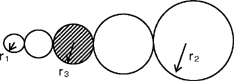# A geometry problem by Tushar Verma

Geometry Level 3The five circles shown above have two direct common tangents. If $r_1 = 8$ cm, $r_2 = 50$ cm, find $r_3$.

×